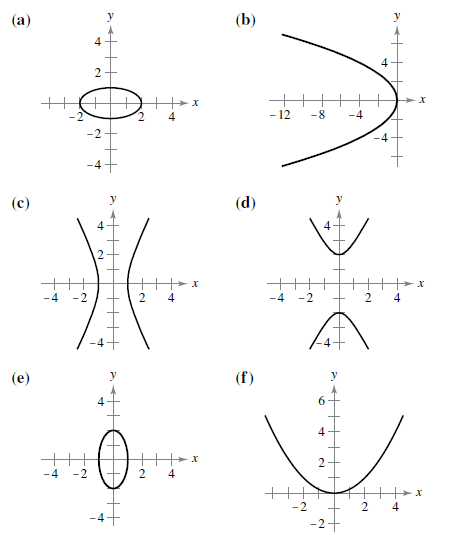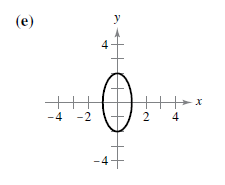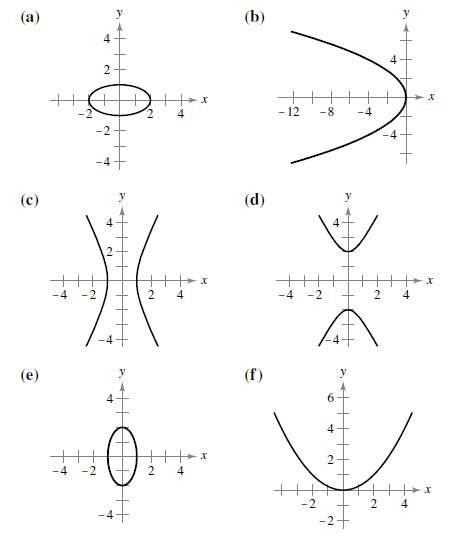Chapter 10, Problem 1RE

Chapter
Section
Textbook Problem

# Matching In Exercises 1-6, match the equation with its graph. [The graphs are labeled (a), (b), (c), (d), (e), and (f).]To determine

If the equation 4x2+y2=4 matches with its graph:Solution:

The equation 4x2+y2=4 matches with graph (e) and the graph isExplanation

Given:

The options are:Explanation:

Here, x and y both are squared terms and both of their coefficients are positive. Thus, this equation represents an ellipse.

Now, in order to find whether the ellipse is horizontal or vertical, represent the equation in the standard form of an ellipse.

The given equation is:

4x2+y2=4

Divide the equation by 4:

x2+14y2=1

Rearrange it in standard form as:

x212+y222=1

So,

(xh)2b2+(yk)2a2=1

Which is the standard equation for an ellipse, whose major axis is vertical. That is the center of ellipse is (h,k)=(0,0) with semi axis a=1,b=2, focus is (0,3) and vertex be (0,2)

Thus, the correct graph matches for the equation that holds the above properties is option (e).

#### The Solution to Your Study Problems

Bartleby provides explanations to thousands of textbook problems written by our experts, many with advanced degrees!

Get Started

#### Solve the equations in Exercises 126. x1x=0

Finite Mathematics and Applied Calculus (MindTap Course List)

#### In the problems 7-8, find the intercept and graph the functions 5x6y=30

Mathematical Applications for the Management, Life, and Social Sciences

#### If sin =12, find: a csc b

Elementary Geometry For College Students, 7e

#### Evaluate .

Study Guide for Stewart's Single Variable Calculus: Early Transcendentals, 8th

#### Explain the role of voluntary participation in informed consent.

Research Methods for the Behavioral Sciences (MindTap Course List)